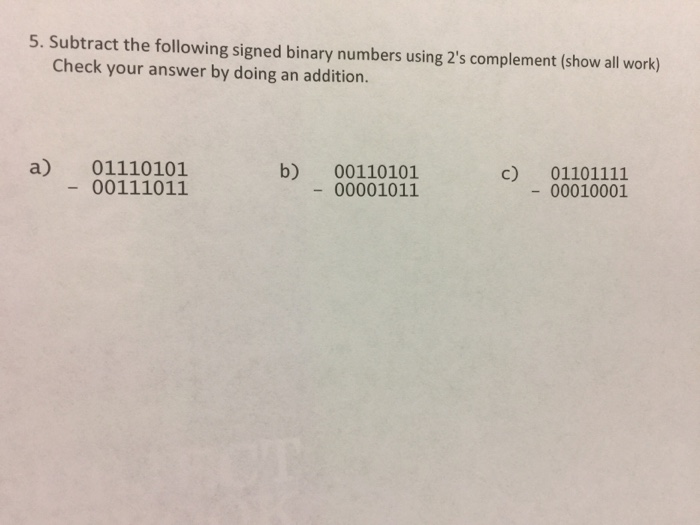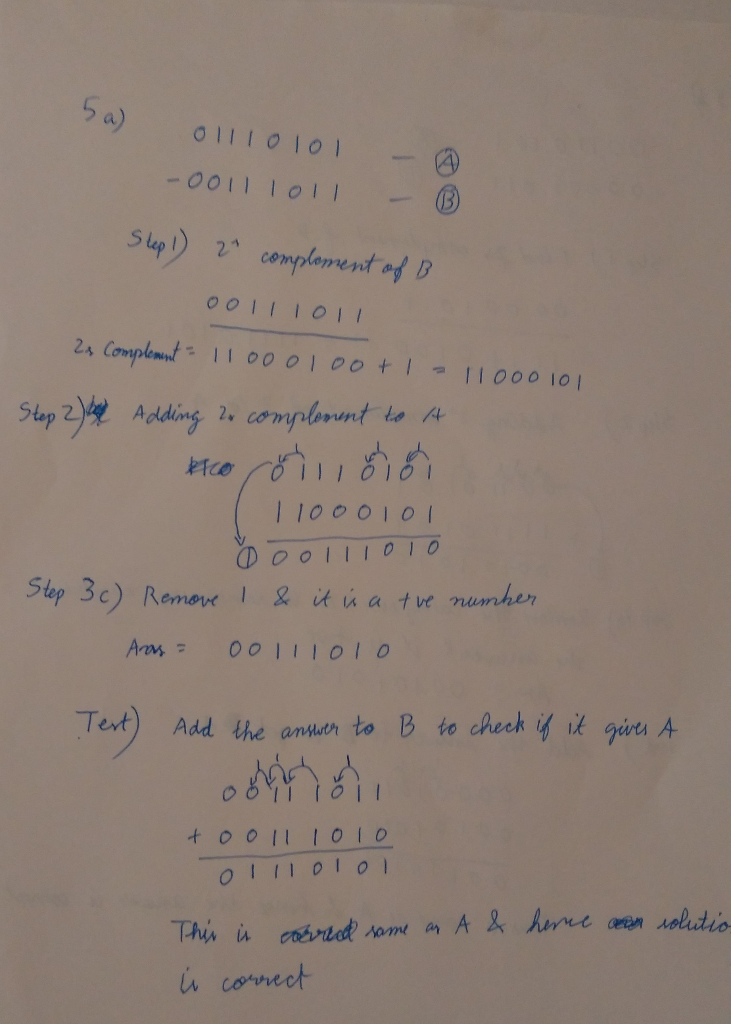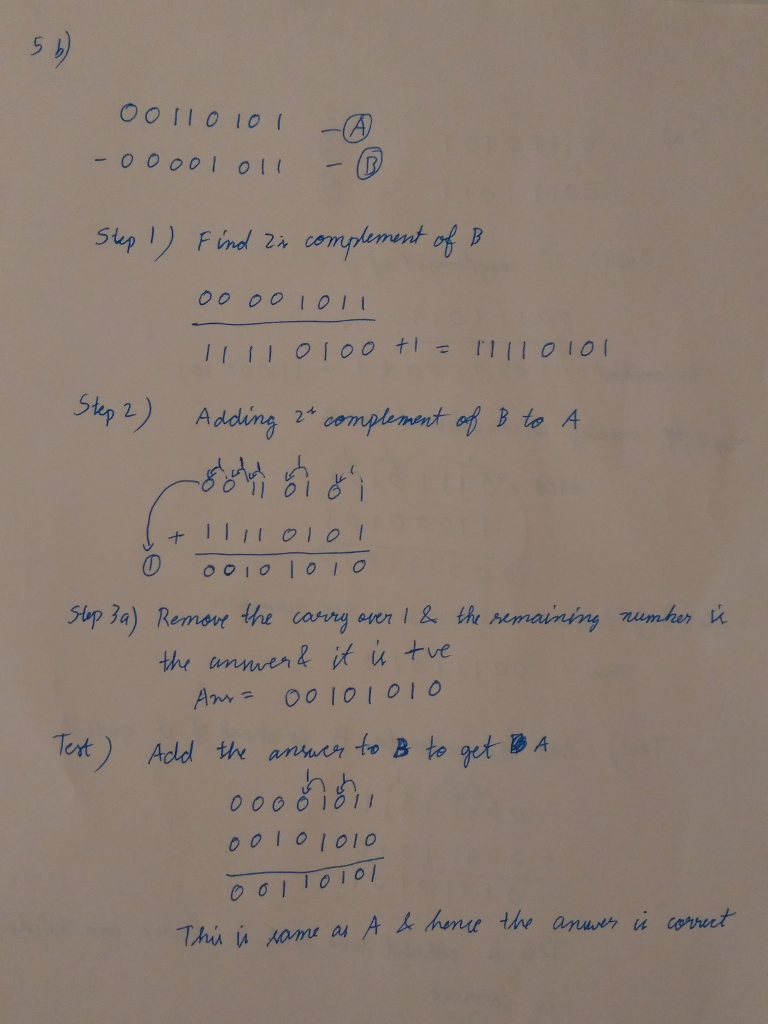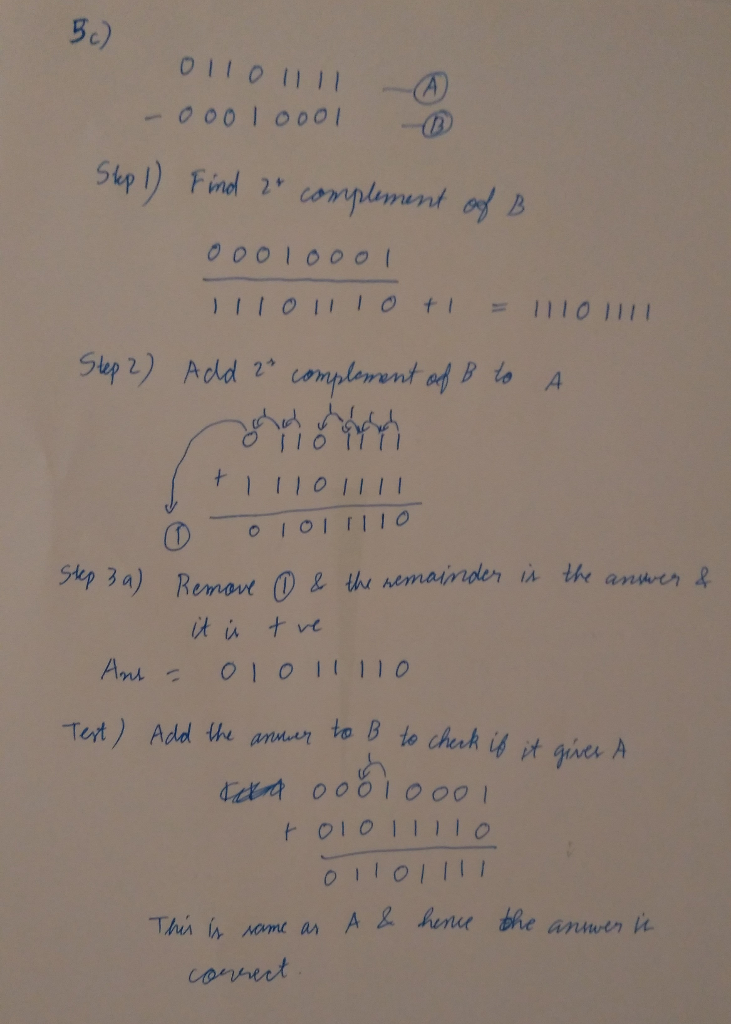# Question & Answer: Subtract the following signed binary numbers using 2's complement (show all work) Check your answer b…..Subtract the following signed binary numbers using 2’s complement (show all work) Check your answer by doing an addition. a) 01110101 -00111011 b) 00110101 -00001011 c) 01101111 – 00010001

The following steps are done to subtract number A from number B:

Don't use plagiarized sources. Get Your Custom Essay on
Question & Answer: Subtract the following signed binary numbers using 2's complement (show all work) Check your answer b…..
GET AN ESSAY WRITTEN FOR YOU FROM AS LOW AS \$13/PAGE

1. At first, 2’s complement of the the number to be subtracted(B) is found. This is done by inverting 1s to 0s and vice versa(1’s complement) and adding 1 to it

2. Then it is added to the number from which subtraction is to be done(A).

3a. If the final carry over of the sum is 1, it is dropped and the result is positive.

3b. If there is no carry over, the two’s complement of the sum will be the result and it is negative.

Test. Then we will test the same by adding the answer to B. If the result of that addition is same as A, the answer is verified

The work for the following is shown in the snapshots: